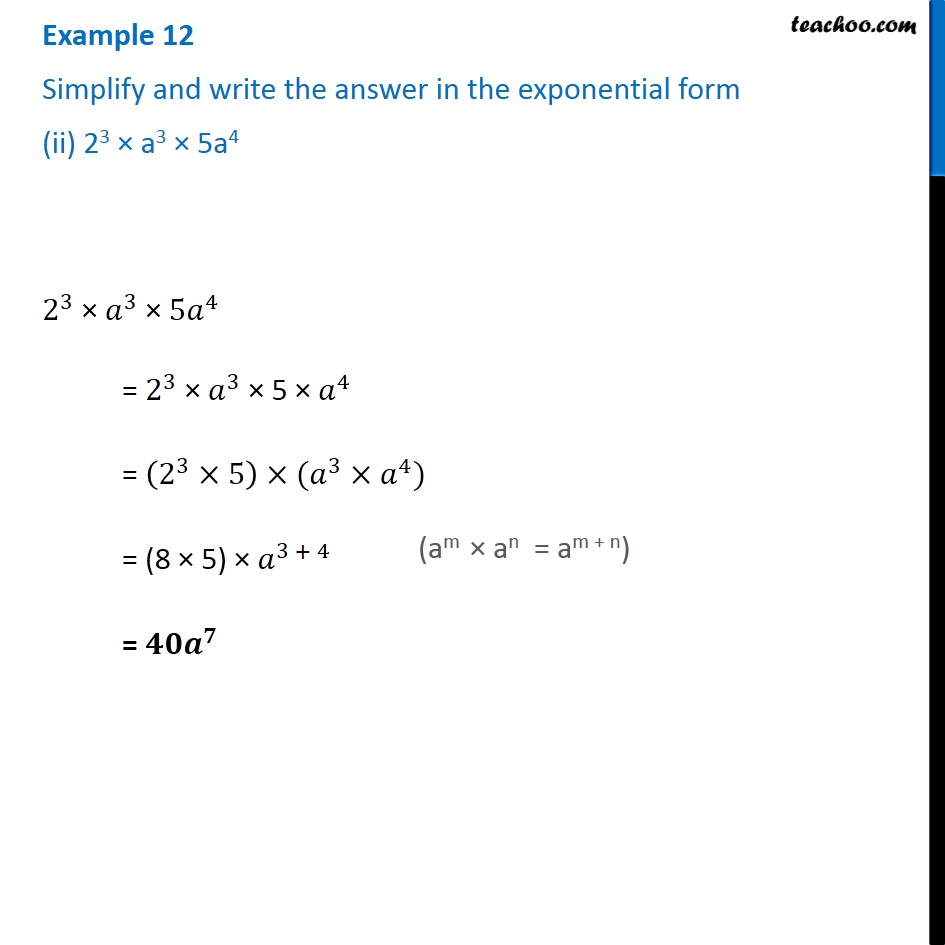1. Chapter 13 Class 7 Exponents and Powers
2. Serial order wise
3. Examples

Transcript

Example 12 Simplify and write the answer in the exponential form (ii) 23 × a3 × 5a4 2^3 × 𝑎^3 × 〖5𝑎〗^4 = 2^3 × 𝑎^3 × 5 × 𝑎^4 = (2^3×5)×(𝑎^3×𝑎^4) = (8 × 5) × 𝑎^(3 + 4) = 〖𝟒𝟎𝒂〗^𝟕 (am × an = am + n)